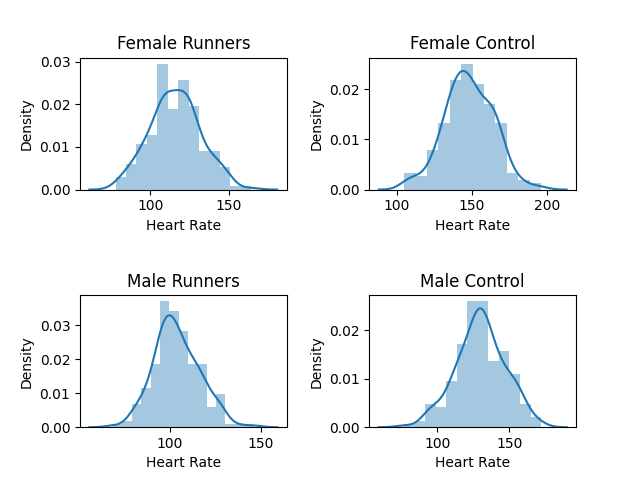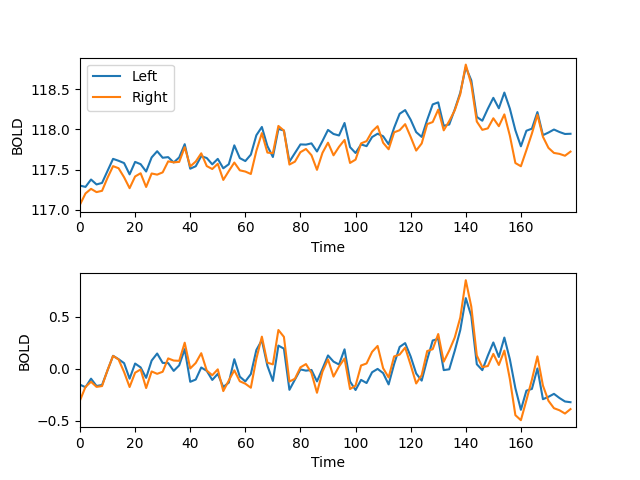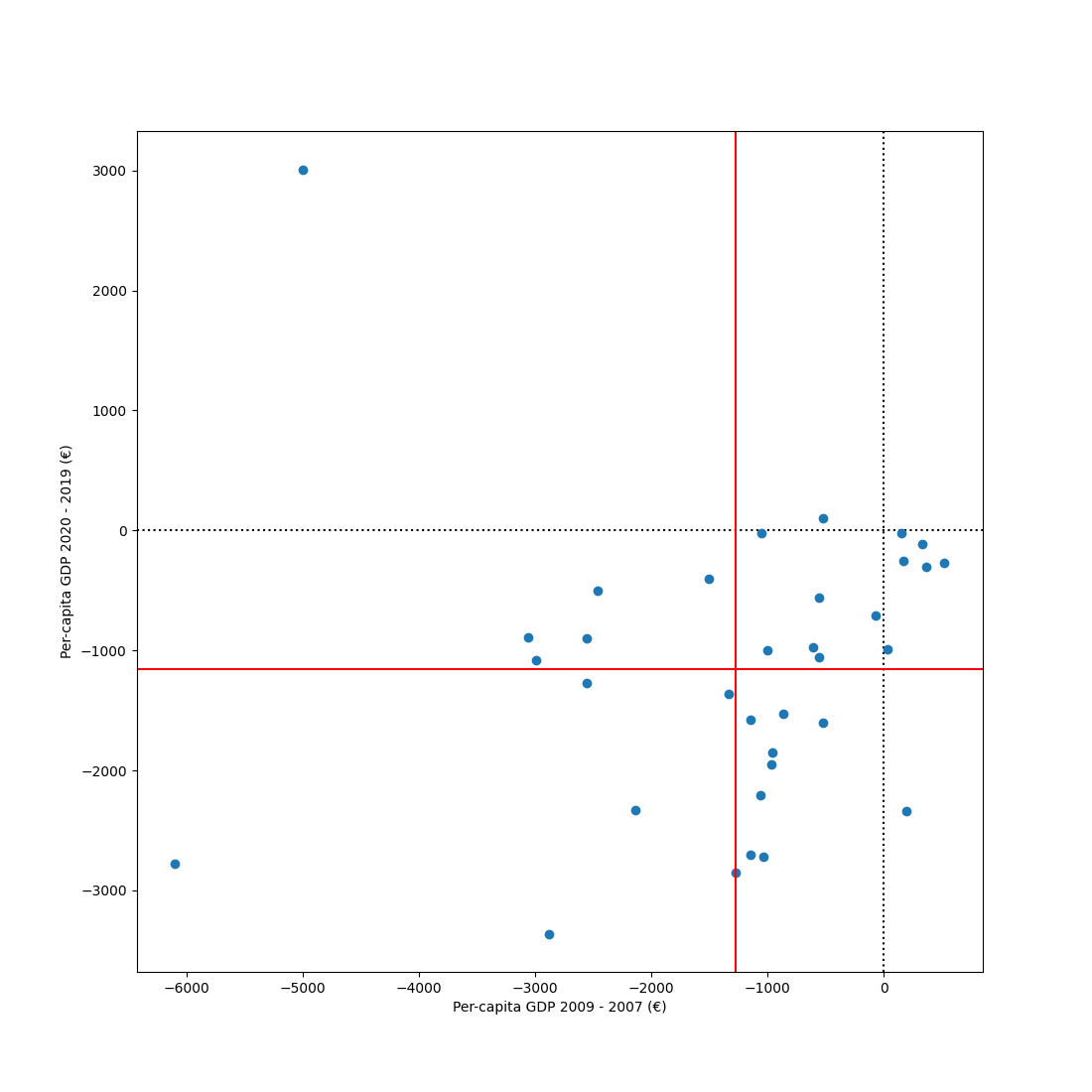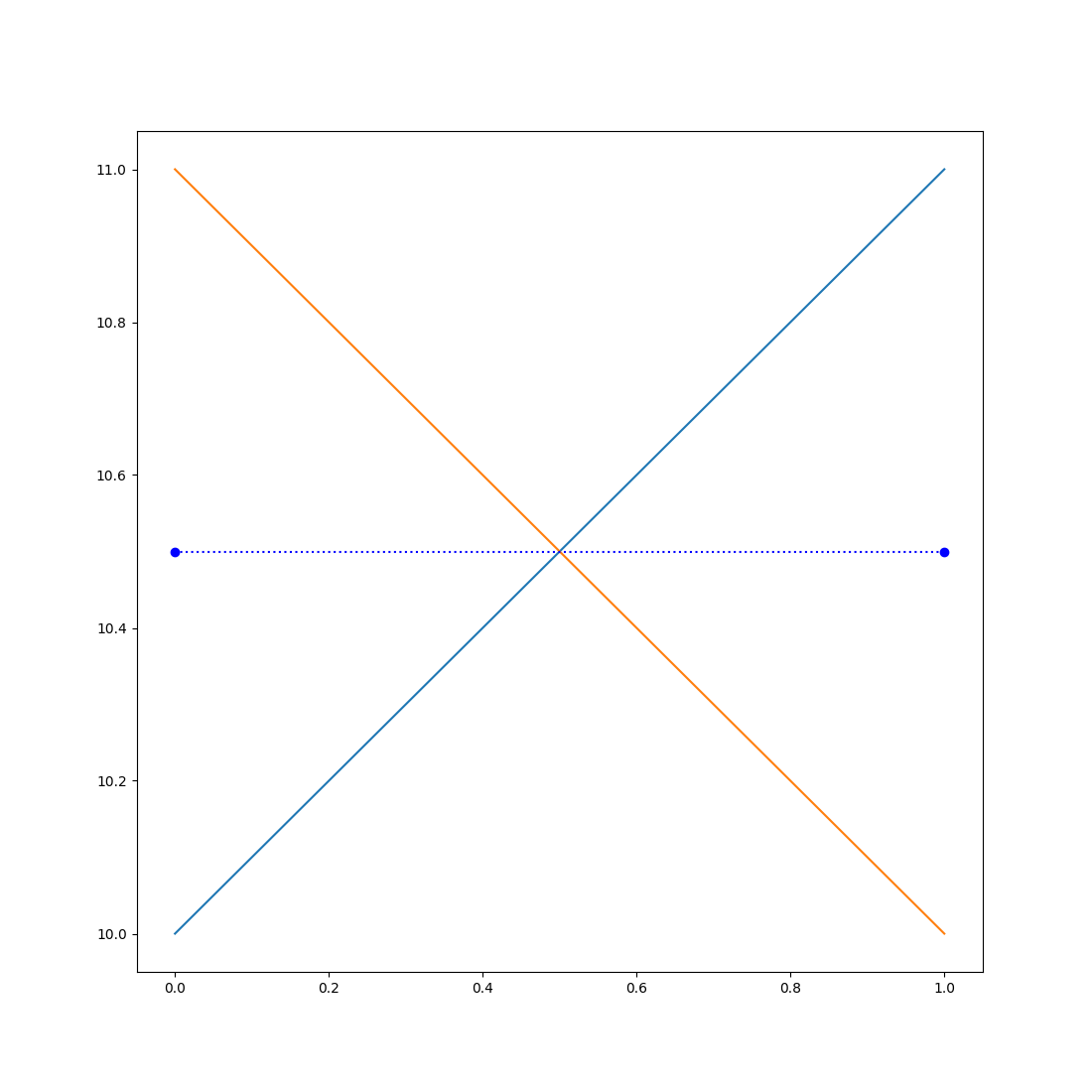# Plotting with Seaborn and Maplotlib

## Libraries for plotting

The traditional Python library for plotting (or data visualization) is Matplotlib. This is a comprehensive library that allows you to create any kind of plot that you can think of. However, Matplotlib can be cumbersome to use. Therefore, Seaborn was built on top of Matplotlib to make it easier to create common plot types, such as bar plots, or line plots (which Seaborn calls 'point plots').

## Plotting simple lines and dots

It is convention to import `matplotlib.pyplot` as `plt`. This is the module that contains most of the plotting functions. The main plotting function is `plt.plot()`. You can call `plt.plot()` multiple times, and then call `plt.show()` to show the resulting plot. If you do this from a code editor that supports this, such as Rapunzel or Spyder, the plot will be shown in the interactive console. Otherwise the plot will pop up in a separate window.

```from matplotlib import pyplot as plt

# Simplest case: only specify Y values
plt.plot([10, 11])
# Specfy both X and Y values
plt.plot([0, 1], [11, 10])
# Also specify a line format (circles with dotted lines) and a color
plt.plot([0, 1], [10.5, 10.5], 'o:', color='blue')
plt.show()
```There are many functions to make your plot look better, for example by adding labels to the axes. You can find a list of all functions here.

Let's consider a slightly more realistic example: a scatterplot of movie ratings over the years, based on this data.

```from datamatrix import io

# The ',' format indicates tiny markers without lines
plt.plot(dm.year, dm.rating, ',')
plt.xlabel('Year')
plt.ylabel('Rating')
plt.title('Movie ratings over the years')
plt.show()
```## Creating common plots

### Point plot (also: line plot)

It is convention to import `seaborn` as `sns`. Seaborn is not as powerful as Matplotlib, but it has several convenient functions for creating common plot types. Often you will still use Matplotlib in addition to Seaborn to perfect your plot, for example by adding labels.

Most Seaborn functions, including `sns.pointplot()`, take a DataMatrix or DataFrame object with the `data` keyword. You also need to specify which columns should be used for the X and the Y axes. You can optionally specify a `hue` keyword (not used here), which specifies a column that should be used to draw differently colored lines (in the case of a point plot), differently colored bars (in the case of a bar plot), etc.

```import seaborn as sns

dm90s = (dm.year > 1990) & (dm.year < 2000)
sns.pointplot(x='year', y='rating', data=dm90s)
plt.xlabel('Year')
plt.ylabel('Rating')
plt.show()
```### Bar plot

Let's again consider this dataset from Moore, McCabe, & Craig (included as example data with JASP). This data contains the heart rate of male and female runners and control participants. So let's plot heart rate as a function of Gender (X axis) and Group (hue).

```dm = io.readtxt('data/heartrate.csv')
sns.barplot(
x='Gender',
y='Heart Rate',
hue='Group',
data=dm
)
plt.xlabel('Gender')
plt.ylabel('Heart rate')
plt.show()
```### Distribution plot (also: histogram)

The plots above show average values. It's also important to have a sense of how data is distributed. Creating a distribution plot is very easy with Seaborn. (Incidentally, this example also show how you can refer to column names that contains spaces, special characters, etc.: with `dm['column_name']` instead of the normal `dm.column_name` syntax.)

```sns.distplot(dm['Heart Rate'])
plt.show()
```## Creating subplots

You can create subplots with `plt.subplot()`. This takes a number of rows, a number of columns, and then the number of the subplot, where subplots are numbered from left to right and then from top to bottom. So if you have 3 (rows) x 3 (columns) plot, then subplot 4 would be the first subplot on the middle row.

You can use `plt.subplots_adjust()` to add some spacing between the rows (`hspace`) and the columns (`wspace`).

```from datamatrix import operations as ops

dm_female, dm_male = ops.split(dm.Gender, 'Female', 'Male')
plt.subplot(1, 2, 1)
plt.title('Women')
sns.barplot(x='Group', y='Heart Rate', data=dm_female)
plt.ylim(90, 160)
plt.xlabel('Group')
plt.ylabel('Heart rate')
plt.subplot(1, 2, 2)
plt.title('Men')
sns.barplot(x='Group', y='Heart Rate', data=dm_male)
plt.ylim(90, 160)
plt.xlabel('Group')
plt.ylabel('Heart rate')
plt.show()
```## Exercises

### Plotting rank-ordered ratings for 90s movies

Using this dataset, for each year in the 90s separately, plot the ratings for individual movies, rank-ordered such that the lowest-rated movies start on the left. The resulting plot consists of ten lines (for each year in the 90s) that gradually go up, with each datapoint corresponding to a single movie rating.

View solution

### Plotting hear-rate distributions in subplots

Use this dataset from Moore, McCabe, & Craig to create a two-by-two plot, and in each subplot show the distribution of heart rate for one combination of gender and group of participants (Men Runners, Men Control, Female Runners, Female Control).

View solution